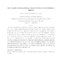## Fast Multipole Accelerated Boundary Element Methods for the 3D Helmholtz Equation2008-01-02
##### Authors
Gumerov, Nail A.
Duraiswami, Ramani
##### Abstract
The development of a fast multipole method accelerated iterative solution of the boundary element equations for large problems involving hundreds of thousands elements for the Helmholtz equations in 3D is described. The BEM requires several approximate computations (numerical quadrature, approximations of the boundary shapes using elements) and the convergence criterion for iterative computation. When accelerated using the FMM, these different errors must all be chosen in a way that on the one hand excess work is not done and on the other that the error achieved by the overall computation is acceptable. Details of translation operators used, choices of representations, and boundary element integration consistent with these approximations are described. A novel preconditioner for accelerating convergence, using a low accuracy FMM accelerated solver as a right preconditioner is also described. Results of the developed and tested solvers for boundary value problems for the Helmholtz equations using the solver are presented for the number of unknowns N <= 106 and product of wavenumber k times domain size D, kD <= 200 and show good performance close to theoretical expectations.